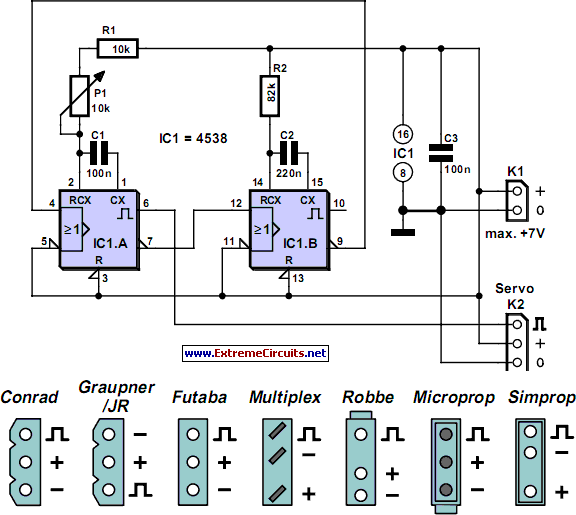# Learning Electronics

Learn to build electronic circuits

# Servo Tester Using A 4538

There are times when a small servo tester for modelling comes in very useful. Everybody who regularly works with servos will know several instances when such a servo tester will come in handy. The function of a servo tester is to generate a pulsing signal where the width of the positive pulse can be varied between 1 and 2 ms. This pulse-width determines the position the servo should move to. The signal has to repeat itself continuously, with a frequency of about 40 to 60 Hz. These circuits often use an NE555 or one of its derivatives to generate the pulses. This time we have used a 4538 for variety. This IC contains two astable multi-vibrators. You can see from the circuit diagram that not many other components are required besides the 4538. The astable multi-vibrator in a 4538 can be started in two ways. When input I 0 (pin 5 or 11) is high, a rising edge on input I 1 (pin 4 or 12) is the start signal to generate a pulse.

Circuit diagram:Servo Tester Using A 4538 Circuit Diagram

The pulse-width at the output of IC1a is equal to (R1+P1)×C1. This means that when potentiometer P1 is turned to its minimum resistance, the pulse-width will be 10 k × 100 n = 1 ms. When P1 is set to maximum (10 k), the pulse-width becomes 20 k × 100 n = 2 ms. At the end of this pulse inverting output Q generates a rising edge. This edge triggers IC1.B, which then generates a pulse. The pulse-width here is 82 k × 220 n ˜ 18 ms. At the end of this pulse the Q output will also generate a rising edge. This in turn makes IC1.A generate a pulse again. This completes the circle. Depending on P1, the total period is between 19 and 20 ms. This corresponds to a frequency of about 50 to 53 Hz and is therefore well within the permitted frequency range.
Author: Paul Goossens - Copyright: Elektor July-August 2004